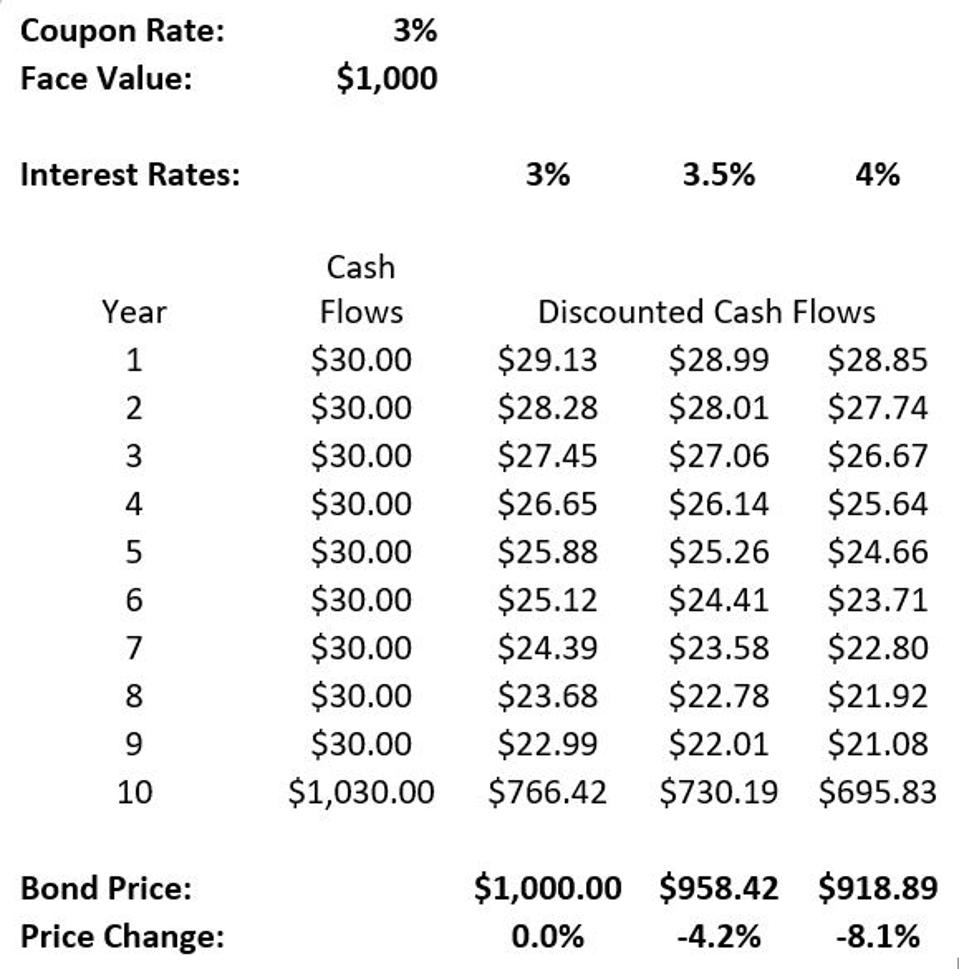# Bond DurationRetirement

Bond prices are sensitive to interest rate changes, and bond duration is a measure of just how sensitive. For instance, in Exhibit 1.1 (shown in my last article), an increase in interest rates for the simple bond from 3 percent to 4 percent caused the bond’s price to fall by 8.1 percent. This bond has a duration of 8.1, meaning that a 1 percent rise in interest rates leads to an 8.1 percent drop in price. The bond duration is measured in years, and the weighted-dollar average for the time when the cash flows are received in this example is 8.1 years.

### Exhibit 1.1 Basic Pricing for a Ten-Year Coupon Bond

Exhibit 1.3 uses the same basic setup as Exhibit 1.1 to provide more insight about how bond prices relate to interest rates and time to maturity. Again, the basic scenario is that we have purchased a bond with a \$1,000 face value and 3 percent annual coupon payments. The current interest rate for a comparable bond is also 3 percent. Sometime shortly after purchase, interest rates change, with the exhibit showing new potential interest rates from 1 to 5 percent. The exhibit shows the new price that bonds with different maturities could sell for after the rate change. The bottom section of the exhibit shows the percentage change in price resulting from the rate change. Here we can clearly see how bond prices move counter to interest rates, and how price fluctuations are more dramatic for longer-term bonds, demonstrating their higher duration. At the extreme, the thirty-year bond would experience a capital gain of 22.4 percent if interest rates fell by 1 percent, and a 17.3 percent capital loss if interest rates rose by 1 percent. Price risk increases with time to maturity. If interest rates rose to 5 percent, the capital loss for a thirty-year bond would be 30.7 percent—comparable to a significant stock market drop. Despite their reputation as reliable and predictable, bonds can be risky.

### Exhibit 1.3 Bond Prices and Interest Rates

While more complex bonds can have some unusual duration properties, the basic noncallable coupon and discount bonds we consider for a retirement income plan define duration in a straightforward way. A bond’s duration is essentially the effective maturity of a bond—an average of when the bond’s payments are received, weighted by the discounted size of those cash flows.

A zero-coupon bond provides one payment at the maturity date, so its duration is the same as the time to maturity. The further away the maturity date, the higher the bond’s duration, making it more sensitive to interest rate changes. A coupon bond will have a shorter duration than the time to the maturity date because coupon payments are received before the maturity date. Higher coupon rates push relatively more cash flows sooner, which otherwise lowers the duration for a bond with the same maturity date. Also, lower interest rates mean the future cash flows from a bond are discounted less relative to nearer-term cash flows, and so bond duration increases when interest rates are low. An implication for this point is that our low-interest-rate environment increases the interest rate risk for holding bonds, as a rate increase can result in a bigger capital loss.

An observant reader of Exhibit 1.3 might note that duration is not symmetric. For a thirty-year bond, a 1 percent increase in interest rates to 4 percent results in a capital loss of 17.3 percent, while a 1 percent decrease in interest rates to 1 percent results in a capital gain of 22.4 percent. The duration measure works best for small interest rate changes because it is a linear approximation to a shape that is curved. The term convexity describes price sensitivity to interest changes more precisely. Bond prices are more sensitive to rate decreases (prices rise more) than to equivalent rate increases (prices fall by less). These differences are accounted for by the fact that changing interest rates also impact duration. The duration for a given bond rises as interest rates fall and future cash flows are discounted by less. But for a household retiree, duration provides a close enough approximation to this relationship, and only those with a greater interest in the mathematics of bond pricing should worry about further adding bond convexity to their discussion.